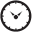# CA Service Management

View Only

## Quering the last 7 days of the activity log via Unix timestamp field [mdb].[dbo].[act_log].[system_time] via Transact-SQL#### STUART MATTHEWSMar 29, 2023 05:15 PM• #### 1.  Quering the last 7 days of the activity log via Unix timestamp field [mdb].[dbo].[act_log].[system_time] via Transact-SQL

Posted Mar 29, 2023 05:15 PM

Hello,

We don't have CABI installed. I want our MSSQL Database Administrators to setup a T-SQL query against the SDM 17.3 request/incident/problem activity log, that runs every monday and sends an email.

Does anyone know how to get the Last 7 days value of [mdb].[dbo].[act_log].[system_time] as its in Unix time? I'm only aware of how to do a basic fixed timestamp query like below:

`/*`
`Search for all activity log entries by the analyst/contact_uid`
`Epoch Converter`

`1679223600 = Monday, 20 March 2023 00:00:00 GMT+13:00 DST`
`1680087600 = Thursday, 30 March 2023 00:00:00 GMT+13:00`

`Count of requests updated */`

`SELECT`
`COUNT (DISTINCT [mdb].[dbo].[call_req].[ref_num])`
`FROM [mdb].[dbo].[call_req]`
`INNER JOIN [mdb].[dbo].[act_log] ON [mdb].[dbo].[call_req].[persid]=[mdb].[dbo].[act_log].[call_req_id]`
`WHERE [mdb].[dbo].[act_log].[analyst]=0xD18B4835EA28A643800260AA1C282A87`
`AND [mdb].[dbo].[act_log].[system_time] > 1679223600 AND [mdb].[dbo].[act_log].[system_time] < 1680087600`
`AND [mdb].[dbo].[call_req].[type]='R'`

• #### 2.  RE: Quering the last 7 days of the activity log via Unix timestamp field [mdb].[dbo].[act_log].[system_time] via Transact-SQL Best Answer

Posted Mar 30, 2023 04:41 AM

Hi Stuart,

You need to calculate the number of seconds from 1970 January 1st to 7 days before Current datetime and compare this number with the act_log system_time.

You use a combination of the following sql functions to obtain this number:

The GETDATE() function returns the current database system date and time, in a 'YYYY-MM-DD hh:mm:ss.mmm' format.

The DATEDIFF() function returns the difference between two dates. Syntax. DATEDIFF(interval, date1, date2).

The CONVERT() function converts a value (of any type) into a specified datatype.

I use the following:
DATEDIFF(S, '19700101 00:00:00:000', CONVERT(DateTime, DATEDIFF(DAY, +7, GETDATE())))

First you need calculate the number of days from 1970 January 1st to 7 days before Current datetime.
Second you need to convert this number of days in a date 'YYYY-MM-DD hh:mm:ss.mmm' format.
Finally you need to calculate the number of seconds from 1970 January 1st to this number of days.

So, you can use it in your select:
SELECT
COUNT (DISTINCT [mdb].[dbo].[call_req].[ref_num])
FROM [mdb].[dbo].[call_req]
INNER JOIN [mdb].[dbo].[act_log] ON [mdb].[dbo].[call_req].[persid]=[mdb].[dbo].[act_log].[call_req_id]
WHERE [mdb].[dbo].[act_log].[analyst]=0xD18B4835EA28A643800260AA1C282A87
AND [mdb].[dbo].[act_log].[system_time] > DATEDIFF(S, '19700101 00:00:00:000', CONVERT(DateTime, DATEDIFF(DAY, +7, GETDATE())))
AND [mdb].[dbo].[call_req].[type]='R'

Regards,

Alessandro